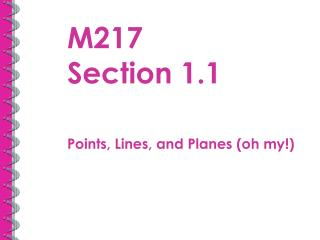DownloadDownload PresentationM217 Section 1.1

# M217 Section 1.1

Télécharger la présentation## M217 Section 1.1

- - - - - - - - - - - - - - - - - - - - - - - - - - - E N D - - - - - - - - - - - - - - - - - - - - - - - - - - -
##### Presentation Transcript

1. M217 Section 1.1 Points, Lines, and Planes (oh my!)

2. Definitions

3. Definitions

4. Example 1 • Name 3 collinear points • Name 3 non-collinear points • List two opposite rays • Are and the same? • List 3 coplanar points

5. Example 2 • Name a point coplanar with • J, K, L • M, N, O • J, Q • Where do segments JQ and QN intersect? • Where do planes JQN and MNO intersect?

6. Point Line and Plane Postulates • A postulate is a statement that is accepted without proof. • Through any 2 pts there is exactly 1 line • Through any 3 noncollinear pts there is exactly one plane containing them • When two lines intersect, the intersection is a point • When two planes intersect, the intersection is a line

7. Drawing Planes • Plane G containing two lines that intersect at point J • Two planes that intersect at line SD